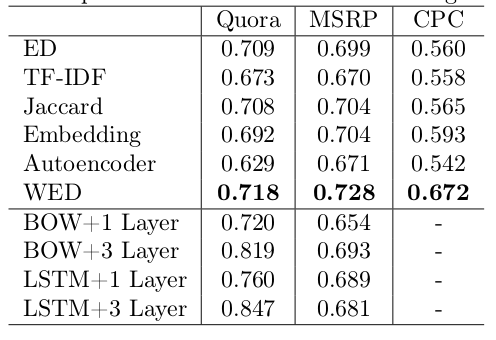# 论文笔记：Word Embedding based Edit Distance

## 作者

Yilin Niu, Chao Qiao, Hang Li, and Minlie Huang

## 观点

• 近年来深度学习被广泛应用到文本相似计算中，但这些方法一般都需要在标注数据上做有监督训练，而标注数据的收集、构建的代码是很高的
• 在特定条件下，WED 可以退化为编辑距离或者 jaccard 系数的变种

One can easily verify that WED degenerates to ED and a variant of Jaccard under certain condition.


不过论文并没有细说这些方法之间是怎么转换的。

## 数据集

• Quora: The dataset released from Quora contains 400k question pairs in community questionanswering labeled as matched or not-matched, 40w 样本
• MSRP: The dataset for paraphrase detection released from Microsoft Research, 5.8k 句子对
• CPC: The dataset referred to as Crowdsourced Paraphrase Collection (CPC), 2.6k 对复述句子

## 模型/方法/结论

$\begin{eqnarray}c_{i,j} = \begin{cases} c_{i,j-1}+i(w_{B}^{j}) \\ c_{i-1,j}+d(w_{A}^{i})\\ c_{i-1,j-1}+s(w_{A}^{i}, w_{B}^{j}) \end{cases}\end{eqnarray}$

$$s(w_{A}^{i}, w_{B}^{j})$$ 则表示将 $$w_{A}^{i}$$ 替换为 $$w_{B}^{j}$$ 的代价，当两者想等时代码就是 0 ，当两者不相等时这个代价就要大于 0。替换操作在不同的地方使用的代价会不一样，有些地方当作和插入、删除一样，即 1，本文的方法则是将替换视作先删除后插入两次操作，所以代价是 2。

$i(w_{B}^{j})=1 - \lambda \cdot max_{w_{A}^{k}\in S_{A}, w_{A}^{k} \neq w_{A}^{i}} (sim(w_{A}^{k}, w_{B}^{j}) + \mu)$

$d(w_{A}^{i})=1 - \lambda \cdot max_{w_{B}^{k}\in S_{B}, w_{B}^{k} \neq w_{B}^{j}} (sim(w_{A}^{i}, w_{B}^{k}) + \mu)$

$s(w_{A}^{i}, w_{B}^{j})=2 - 2 sim(w_{A}^{i}, w_{B}^{j})$

• 当插入新的词时，如果词和句子 A 中的词有相似的，那么对语义的影响就不是那么大
• 当删除句子 A 中的词时，如果句子 B 中还有词和这个删除的词相似，那么对语义的影响也不会那么大

• ED: 经典编辑距离
• TF-IDF: 基于 tfidf 的 cosine 相似
• Embedding: 基于 embedding 的 cosine 相似
• Jaccard: jaccard 系数
• Autoencoder: 这个应该是指语言模型一类的，但是为什么引用的是 Hinton 2006 年的论文……
• BOW+MLP: 有监督方法，给定两个句子，先将句子中的 embedding 加和，然后拼接起来输入到 MLP

分两种情况：一层 MLP 和三层 MLP

• LSTM+MLP: 用 LSTM 编码句子，然后拼接起来输入到 MLP

分两种情况：一层 MLP 和三层 MLP• 本文的方法要优于这几种无监督方法：编辑距离、基于TFIDF的 cosine、基于 word embedding 的 cosine 方法、jaccard 系数
• 对比两个有监督方法(BOW/LSTM+3层MLP)，发现大概在 30k 这个数据量以上，有监督的方法才会显著好于 WED 方法
• 在 Quora 数据集上做了一些采样来分析错误，发现超过 50% 的错误在于没有区分开关键词和非关键词，所以如果有机制能给关键词更高的权重，那么 WED 应该还会有更高的效果 —— 比如说用 TF/IDF 来给每个词加权？

嗯，没有给一些例子呀……

## 相关工作

• Learning semantic representations using convolutional neural networks for web search, 2014
• Convolutional neural network architectures for matching natural language sentences, 2014
• Text matching as image recognition, 2016
• A deep architecture for semantic matching with multiple positional sentence representations, 2016
• A decomposable attention model for natural language inference, 2016
• 编辑距离：Navarro 2001, Jurafsky and Martin 2009
• jaccard 系数：Tan et al 2005
• 基于 word embedding 的 cosine 相似：Mitchell and Lapata 2008, Milajevs et al 2014
• 基于 TFIDF 的 cosine 相似：Buckley, 1988

## 概念和术语

• WED: Word Embedding based Edit Distance 缩写（不应该是 WEED 吗）